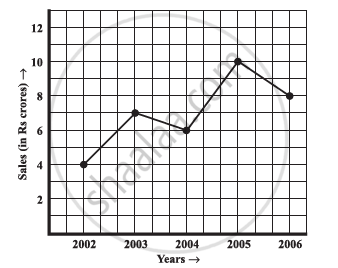# The Following Line Graph Shows the Yearly Sales Figures for a Manufacturing Company.What Were the Sales in (A) 2002 (B) 2006?What Were the Sales in (A) 2003 (B) 2005? - Mathematics

The following line graph shows the yearly sales figures for a manufacturing company.1) What were the sales in (a) 2002 (b) 2006?

2) What were the sales in (a) 2003 (b) 2005?

3) Compute the difference between the sales in 2002 and 2006.

4) In which year was there the greatest difference between the sales as compared
to its previous year?

#### Solution

1)

a. In 2002, the sales were Rs 4 crores.

b. In 2006, the sales were Rs 8 crores.

2)

a) In 2003, the sales were Rs 7 crores.

b) In 2005, the sales were Rs 10 crores

3) In 2002, the sales were Rs 4 crores and in 2006, the sales were Rs 8 crores.

Difference between the sales in 2002 and 2006

= Rs (8 − 4) crores = Rs 4 crores

4) Difference between the sales of the year 2006 and 2005

= Rs (10 − 8) crores = Rs 2 crores

Difference between the sales of the year 2005 and 2004

= Rs (10 − 6) crores = Rs 4 crores

Difference between the sales of the year 2004 and 2003

= Rs (7 − 6) crore = Rs 1 crore

Difference between the sales of the year 2003 and 2002

= Rs (7 − 4) crores = Rs 3 crores

Hence, the difference was the maximum in the year 2005 as compared to its previous year 2004.

Concept: Concept of a Line Graph
Is there an error in this question or solution?

#### APPEARS IN

NCERT Class 8 Maths
Chapter 15 Introduction to Graphs
Exercise 15.1 | Q 2 | Page 237